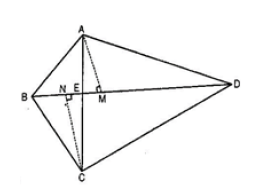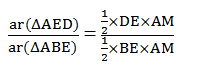Guru

# Diagonals AC and BD of a quadrilateral ABCD intersect each other at P. Show that ar (APB)×ar (CPD) = ar (APD)×ar (BPC). [Hint : From A and C, draw perpendiculars to BD.] Q.6

• 0

Please guide me the best way for solving the question of class 9th math of Areas of Parallelograms and Triangles chapter of exercise 9.4 of math of question no. 6 What is the best way for solving this question, please guide me Diagonals AC and BD of a quadrilateral ABCD intersect each other at P. Show that ar (APB)×ar (CPD) = ar (APD)×ar (BPC). [Hint : From A and C, draw perpendiculars to BD.]

Share

1. Given:

The diagonal AC and BD of the quadrilateral ABCD, intersect each other at point E.

Construction:

From A, draw AM perpendicular to BD

From C, draw CN perpendicular to BDTo Prove,

ar(ΔAED) ar(ΔBEC) = ar (ΔABE) ×ar (ΔCDE)

Proof,

ar(ΔABE) = ½ ×BE×AM………….. (i)

ar(ΔAED) = ½ ×DE×AM………….. (ii)

Dividing eq. ii by i , we get,ar(AED)/ar(ABE) = DE/BE…….. (iii)

Similarly,

ar(CDE)/ar(BEC) = DE/BE ……. (iv)

From eq. (iii) and (iv) , we get

ar(AED)/ar(ABE) = ar(CDE)/ar(BEC)

, ar(ΔAED)×ar(ΔBEC) = ar(ΔABE)×ar (ΔCDE)

Hence proved.

• 0